# Data Structures Lab 8.pptx

mervat32
30 de Mar de 2023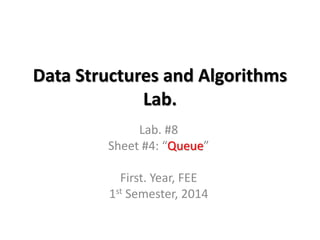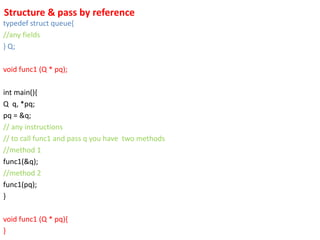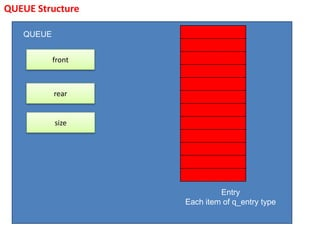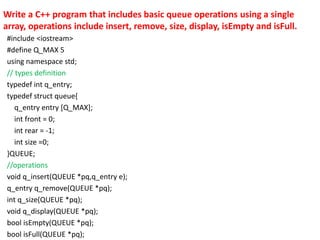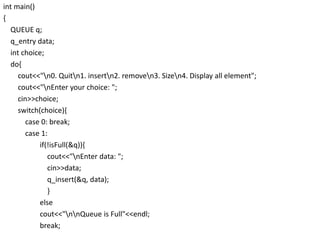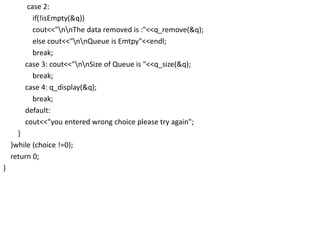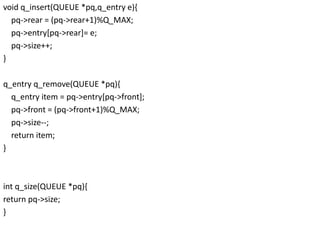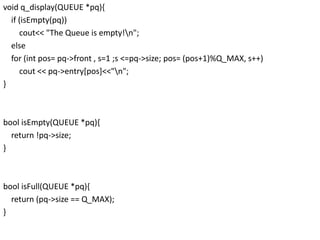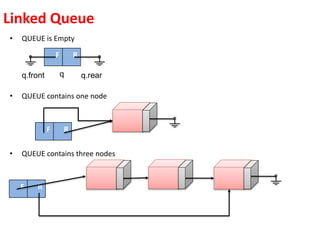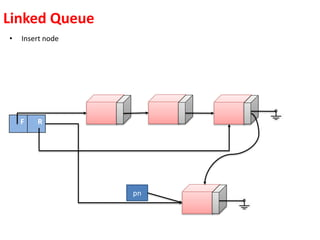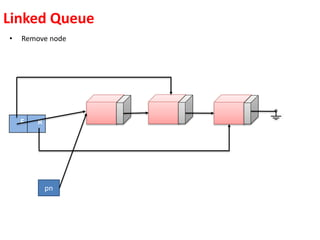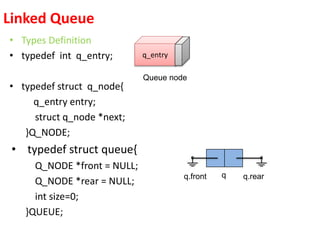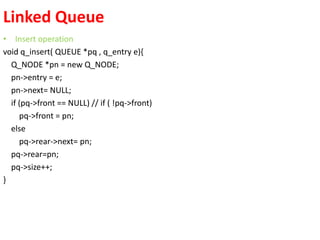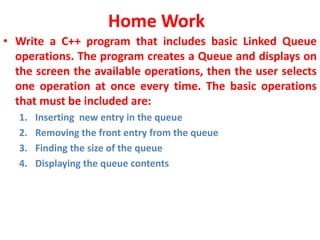1 de 14

### Data Structures Lab 8.pptx

1. Data Structures and Algorithms Lab. Lab. #8 Sheet #4: “Queue” First. Year, FEE 1st Semester, 2014
2. Structure & pass by reference typedef struct queue{ //any fields } Q; void func1 (Q * pq); int main(){ Q q, *pq; pq = &q; // any instructions // to call func1 and pass q you have two methods //method 1 func1(&q); //method 2 func1(pq); } void func1 (Q * pq){ }
3. QUEUE Structure QUEUE Entry Each item of q_entry type front rear size
4. #include <iostream> #define Q_MAX 5 using namespace std; // types definition typedef int q_entry; typedef struct queue{ q_entry entry [Q_MAX]; int front = 0; int rear = -1; int size =0; }QUEUE; //operations void q_insert(QUEUE *pq,q_entry e); q_entry q_remove(QUEUE *pq); int q_size(QUEUE *pq); void q_display(QUEUE *pq); bool isEmpty(QUEUE *pq); bool isFull(QUEUE *pq); Write a C++ program that includes basic queue operations using a single array, operations include insert, remove, size, display, isEmpty and isFull.
5. int main() { QUEUE q; q_entry data; int choice; do{ cout<<"n0. Quitn1. insertn2. removen3. Sizen4. Display all element"; cout<<"nEnter your choice: "; cin>>choice; switch(choice){ case 0: break; case 1: if(!isFull(&q)){ cout<<"nEnter data: "; cin>>data; q_insert(&q, data); } else cout<<"nnQueue is Full"<<endl; break;
6. case 2: if(!isEmpty(&q)) cout<<"nnThe data removed is :"<<q_remove(&q); else cout<<"nnQueue is Emtpy"<<endl; break; case 3: cout<<"nnSize of Queue is "<<q_size(&q); break; case 4: q_display(&q); break; default: cout<<"you entered wrong choice please try again"; } }while (choice !=0); return 0; }
7. void q_insert(QUEUE *pq,q_entry e){ pq->rear = (pq->rear+1)%Q_MAX; pq->entry[pq->rear]= e; pq->size++; } q_entry q_remove(QUEUE *pq){ q_entry item = pq->entry[pq->front]; pq->front = (pq->front+1)%Q_MAX; pq->size--; return item; } int q_size(QUEUE *pq){ return pq->size; }
8. void q_display(QUEUE *pq){ if (isEmpty(pq)) cout<< "The Queue is empty!n"; else for (int pos= pq->front , s=1 ;s <=pq->size; pos= (pos+1)%Q_MAX, s++) cout << pq->entry[pos]<<"n"; } bool isEmpty(QUEUE *pq){ return !pq->size; } bool isFull(QUEUE *pq){ return (pq->size == Q_MAX); }
9. • QUEUE is Empty • QUEUE contains one node • QUEUE contains three nodes Linked Queue R F q q.front q.rear R F R F
10. • Insert node Linked Queue R F pn
11. • Remove node Linked Queue R F pn
12. • Types Definition • typedef int q_entry; • typedef struct q_node{ q_entry entry; struct q_node *next; }Q_NODE; • typedef struct queue{ Q_NODE *front = NULL; Q_NODE *rear = NULL; int size=0; }QUEUE; Linked Queue q_entry Queue node q q.front q.rear
13. • Insert operation void q_insert( QUEUE *pq , q_entry e){ Q_NODE *pn = new Q_NODE; pn->entry = e; pn->next= NULL; if (pq->front == NULL) // if ( !pq->front) pq->front = pn; else pq->rear->next= pn; pq->rear=pn; pq->size++; } Linked Queue
14. Home Work • Write a C++ program that includes basic Linked Queue operations. The program creates a Queue and displays on the screen the available operations, then the user selects one operation at once every time. The basic operations that must be included are: 1. Inserting new entry in the queue 2. Removing the front entry from the queue 3. Finding the size of the queue 4. Displaying the queue contents